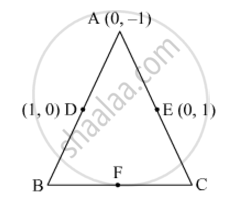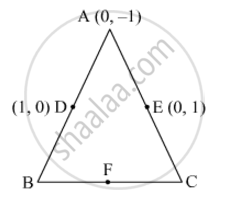# In Fig. 6, ABC is a triangle coordinates of whose vertex A are (0, −1). D and E respectively are the mid-points of the sides AB and AC and their coordinates are (1, 0) and (0, 1) respectively. If F is the mid-point of BC, find the areas of ∆ABC and ∆DEF. - Mathematics

In Fig. 6, ABC is a triangle coordinates of whose vertex A are (0, −1). D and E respectively are the mid-points of the sides AB and AC and their coordinates are (1, 0) and (0, 1) respectively. If F is the mid-point of BC, find the areas of ∆ABC and ∆DEF.#### SolutionLet the coordinates of B and C be (x2, y2) and (x3, y3), respectively.

D is the midpoint of AB.

So,

(1,0)=((x_2+0)/2,(y_2-1)/2)

=>1 = x_2/2

⇒ x2 2 and y2 1

Thus, the coordinates of B are (2, 1).

Similarly, E is the midpoint of AC.

So,

(0,1)=((x_3+0)/2, (y_3-1)/2)

=>0=x_3/2

⇒ x3 0 and y3 3

Thus, the coordinates of C are (0, 3).

Also, F is the midpoint of BC. So, its coordinates are

((2+0)/2,(1+3)/2)=(1,2)

Now,

Area of a triangle = 1/2[x1(y2y3)+x2(y3y1)+x3(y1y2)]

Thus, the area of ABC is

1/2[0(1-3)+2(3+1)+0(-1-1)]

=1/2xx8

=4 square units

And the area of DEF is

1/2[1(12)+0(20)+1(01)]

=1/2xx(-2)

=1 square unit     (Taking the numerical value, as the area cannot be negative)

Concept: Area of a Triangle
Is there an error in this question or solution?
2015-2016 (March) Delhi Set 1
Share